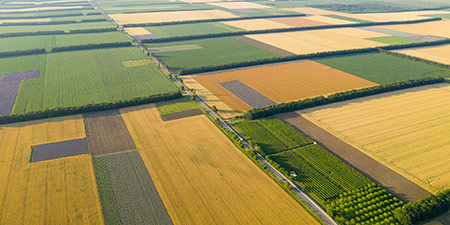# Acre (ac) to Square Foot (ft²)

Area Units ﹣ Converter Acre to Square Foot

Here you can convert the Area unit Acre into the unit Square Foot and vice versa you can convert Square Foot into Acre. By clicking the "Swap units" icon, you will always obtain the desired conversion in the calculation result, i.e., ac to ft² or ft² to ac. With the following calculator you can also calculate any other Area unit.

An acre (ac) is equal to one 160th part of a quarter section or one 640th part of a square mile. Thus, the acre has the area of a square with a side length of about 63.62 meters.

1 ac ≈ 63,62 m × 63,62 m

The unit acre (Old English æcer acre, field) originally referred to a strip of land that could be plowed in one day with a team of oxen.

The unit of area known as the acre is now a common unit for subdividing land areas, especially in America. These are initially divided into square miles (sections) and into quarters of these sections, namely quarters. A quarter section, in turn, consists of 160 acres. In the Anglo-American world, the size of properties in land and property surveys is indicated solely by the two area units acre and square foot.

1/640 square mile = 1/160 quarter = 1 acre = 4.840 square yards = 43.560 square feet = 6.272.640 square inches = 4.046,85642 m²

A square foot (ft²; plural square feet) is equal to the area of a square with a side length of one foot (30.48 cm).

1 ft² = 1 ft × 1 ft

1 ft² = 30,48 cm × 30,48 cm

A foot is 12 inches long in the Anglo-American system and thus a square foot has 144 square inches. In the Anglo-American world, the size of land is indicated in land and property surveys using only the two area units, acre and square foot.

## Basis for conversion Acre (ac) to Square Foot (ft²) and vice versa

The abbreviation for the "Area unit Acre" is ac. The abbreviation for the "Area unit Square Foot" is ft².

## Formula for the conversion of Acre (ac) to Square Foot (ft²) and vice versa

The calculation from Acre to Square Foot shall be made using the following conversion formula:

Conversion formula Acre to Square Foot

Determine the number of Square Foot from Acre

Acre × 43560

## Formula for the conversion of Square Foot (ft²) to Acre (ac)

The calculation from Square Foot to Acre shall be made using the following conversion formula:

Conversion formula Square Foot to Acre

Determine the number of Acre from Square Foot

Square Foot × 2.2956841138659E-5

## Overview table : How many Acre are in a Square Foot ?

Acre ac ⇒ Square Foot ft²
0.01 ac  are  435.60000 ft²
0.02 ac  are  871.20000 ft²
0.03 ac  are  1 306.80000 ft²
0.04 ac  are  1 742.40000 ft²
0.05 ac  are  2 178 ft²
0.06 ac  are  2 613.60000 ft²
0.07 ac  are  3 049.20000 ft²
0.08 ac  are  3 484.80000 ft²
0.09 ac  are  3 920.40000 ft²
0.10 ac  are  4 356 ft²
0.20 ac  are  8 712 ft²
0.30 ac  are  13 068 ft²
0.40 ac  are  17 424 ft²
0.50 ac  are  21 780 ft²
0.60 ac  are  26 136 ft²
0.70 ac  are  30 492 ft²
0.80 ac  are  34 848 ft²
0.90 ac  are  39 204 ft²
1 ac  corresponds to  43 560 ft²
2 ac  are  87 120 ft²
3 ac  are  130 680 ft²
4 ac  are  174 240 ft²
5 ac  are  217 800 ft²
6 ac  are  261 360 ft²
7 ac  are  304 920 ft²
8 ac  are  348 480 ft²
9 ac  are  392 040 ft²
10 ac  are  435 600 ft²
20 ac  are  871 200 ft²
30 ac  are  1 306 800 ft²
40 ac  are  1 742 400 ft²
50 ac  are  2 178 000 ft²
60 ac  are  2 613 600 ft²
70 ac  are  3 049 200 ft²
80 ac  are  3 484 800 ft²
90 ac  are  3 920 400 ft²
100 ac  are  4 356 000 ft²
200 ac  are  8 712 000 ft²
300 ac  are  13 068 000 ft²
400 ac  are  17 424 000 ft²
500 ac  are  21 780 000 ft²
600 ac  are  26 136 000 ft²
700 ac  are  30 492 000 ft²
800 ac  are  34 848 000 ft²
900 ac  are  39 204 000 ft²
1 000 ac  are  43 560 000 ft²

## Overview table : How many Square Foot are in a Acre ?

Square Foot ft² ⇒ Acre ac
0.50 ft²  are  0.00001 ac
0.60 ft²  are  0.00001 ac
0.70 ft²  are  0.00001 ac
0.80 ft²  are  0.00001 ac
0.90 ft²  are  0.00002 ac
1 ft²  corresponds to  0.00002 ac
2 ft²  are  0.00004 ac
3 ft²  are  0.00006 ac
4 ft²  are  0.00009 ac
5 ft²  are  0.00011 ac
6 ft²  are  0.00013 ac
7 ft²  are  0.00016 ac
8 ft²  are  0.00018 ac
9 ft²  are  0.00020 ac
10 ft²  are  0.00023 ac
20 ft²  are  0.00045 ac
30 ft²  are  0.00068 ac
40 ft²  are  0.00091 ac
50 ft²  are  0.00114 ac
60 ft²  are  0.00137 ac
70 ft²  are  0.00160 ac
80 ft²  are  0.00183 ac
90 ft²  are  0.00206 ac
100 ft²  are  0.00229 ac
200 ft²  are  0.00459 ac
300 ft²  are  0.00688 ac
400 ft²  are  0.00918 ac
500 ft²  are  0.01147 ac
600 ft²  are  0.01377 ac
700 ft²  are  0.01607 ac
800 ft²  are  0.01836 ac
900 ft²  are  0.02066 ac
1 000 ft²  are  0.02295 ac

## Source information

As source for the information in the 'Area Units' category, we have used in particular:

## Last update

This page of the 'Area Units' category was last edited or reviewed by Michael Mühl on February 20, 2023. It corresponds to the current status.

### Changes in this category "Area Units"

• Publication of Area Converter together with associated texts.